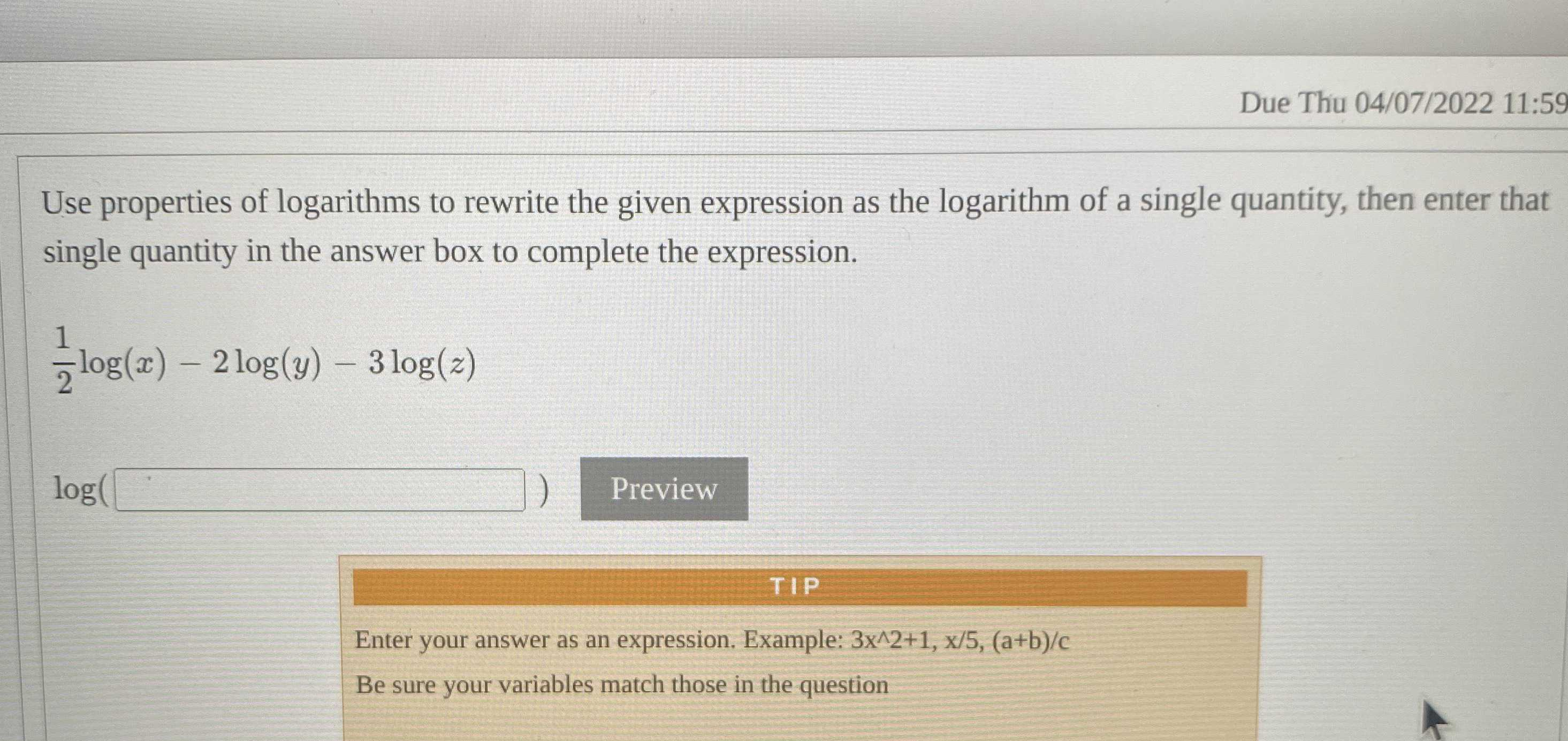### Still have math questions?

Algebra
QuestionUse properties of logarithms to rewrite the given expression as the logarithm of a single quantity, then enter that single quantity in the answer box to complete the expression.

$$\frac { 1 } { 2 } \log ( x ) - 2 \log ( y ) - 3 \log ( z )$$ .

$$\log_{}{\frac{x^{\frac{1}{2} } }{y^{2}z^{3} } }$$